# 不认得各种中药材？来看看飞桨开发者的妙招

2019/12/30 20:00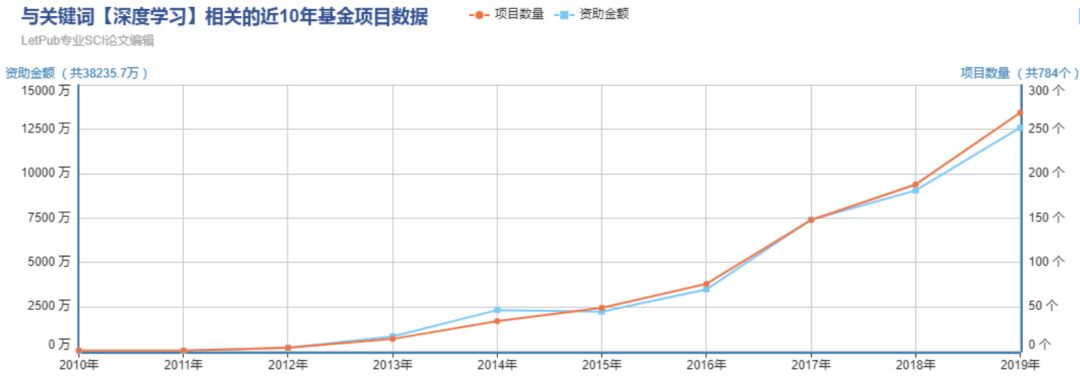# 方案解析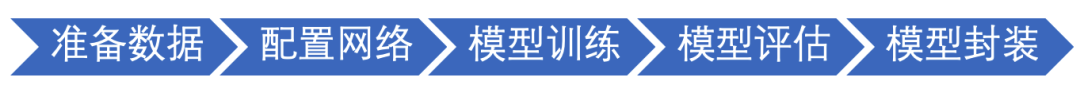01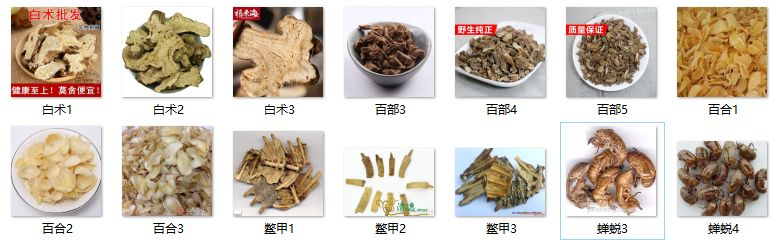02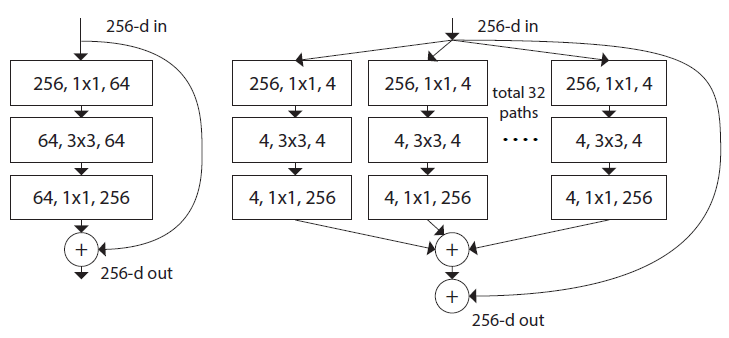Left：ABlock of ResNet

Right：Ablock of ResNeXt with cardinality=32,with roughly the same complexity

def bottleneck_block(self,input,num_filters,stride,cardinality,reduction_ratio,name=None):
conv0 = self.conv_bn_layer(
input=input,
num_filters=num_filters,
filter_size=1,
act='relu',
name='conv' + name + '_x1')
conv1 = self.conv_bn_layer(
input=conv0,
num_filters=num_filters,
filter_size=3,
stride=stride,
groups=cardinality,
act='relu',
name='conv' + name + '_x2')
conv2 = self.conv_bn_layer(
input=conv1,
num_filters=num_filters * 2,
filter_size=1,
act=None,
name='conv' + name + '_x3')
scale = self.squeeze_excitation(
input=conv2,
num_channels=num_filters * 2,
reduction_ratio=reduction_ratio,
name='fc' + name)
short = self.shortcut(input, num_filters * 2, stride, name=name)
return fluid.layers.elementwise_add(x=short, y=scale, act='relu')


ResNeXt-50的整体网络结构如下,主要是将ResNet单元换成了ResNeXt单元。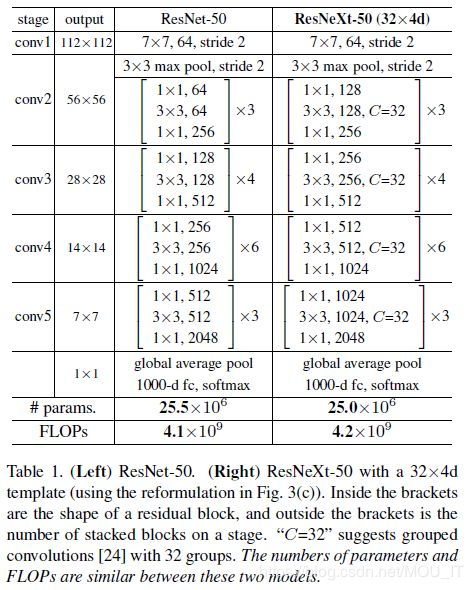Left：ResNet-50

Right：ResNeXt-50with a 32*4d template

03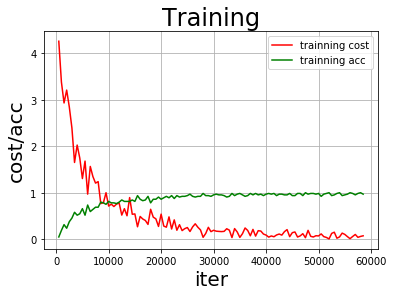04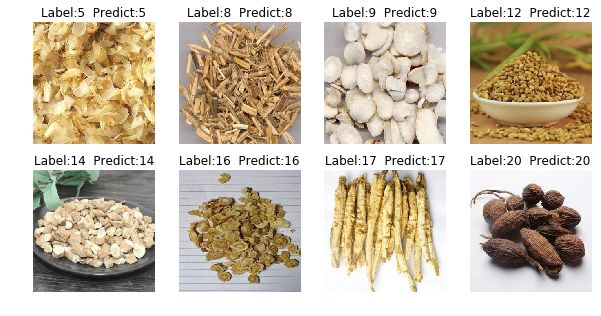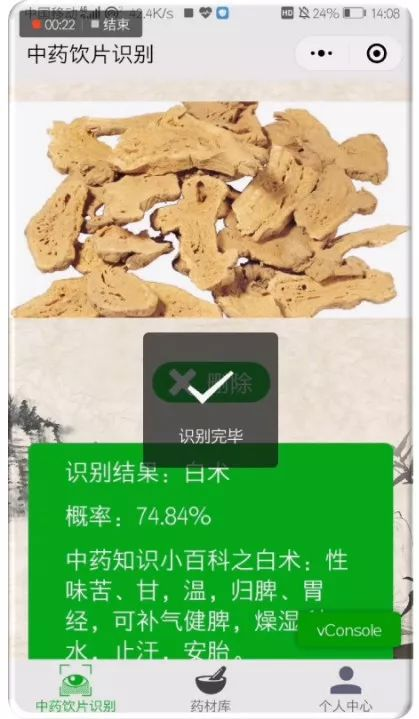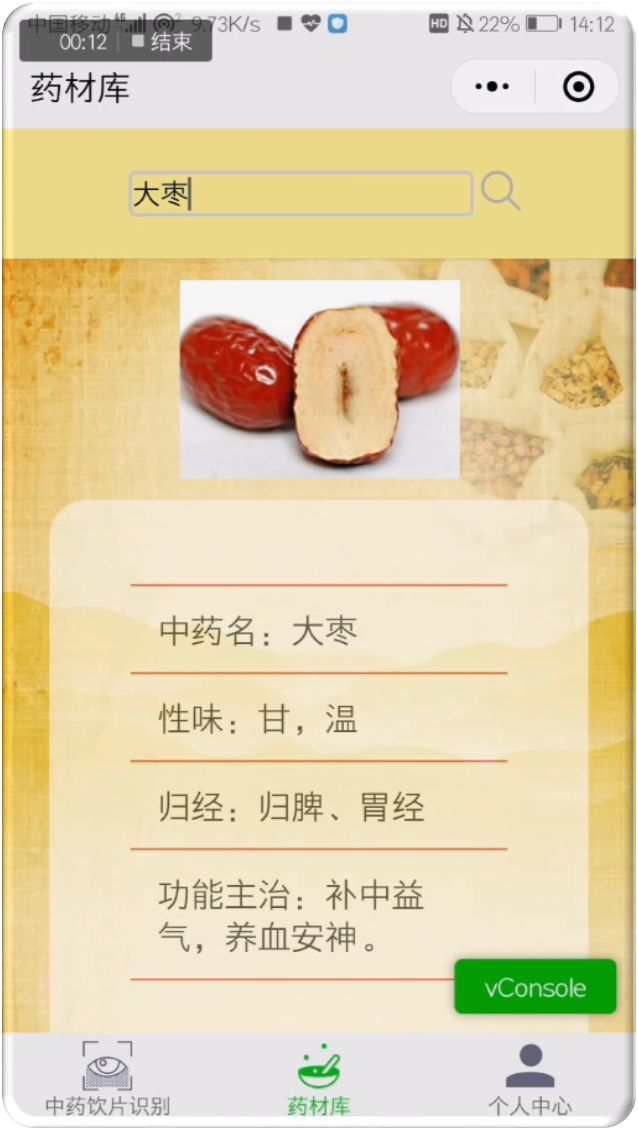05

# 飞桨复现了经典和前沿深度学习算法，快速迭代更新，同步学术前沿，且提供高质量支持迁移学习的工业级预训练模型，利用飞桨进行中药材图片辨识取得较好的识别效果，基于微信小程序的应用也更便于人们使用，结合AI Studio平台的强大算力、丰富的数据、海量开源算法和优质共享项目，为AI技术学习者和行业应用者打开了一扇快速通往人工智能的大门。

GitHub地址：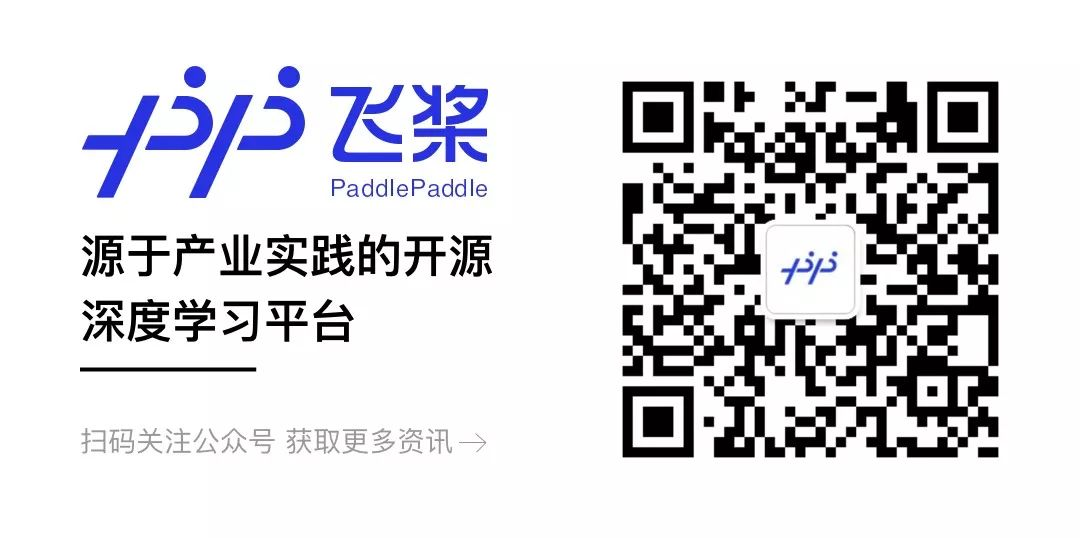### 作者的其它热门文章

0
0 收藏

0 评论
0 收藏
0